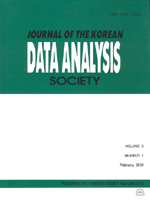상세검색
최근 검색어 전체 삭제
다국어입력
즐겨찾기0KCI등재 학술저널

# Modified Broemeling’s Method Confidence Intervals for the Ratio(C) of Total Variance in Two-Factor Nested Variance Components Model

• 등재여부 : KCI등재
• 2014.06
• 1171 - 1179 (9 pages)

The confidence intervals for σ²C/(σ²A+σ²B+σ²C) are given in various forms by many authors in two-factor nested variance components model Yijk=μ+Ai+Bij+Cijk. In this model, we can find out the confidence intervals for σ²B/σ²C by using Broemeling’s method of the confidence intervals for σ²A/σ²C and σ²A/σ²B. And we can find out a confidence intervals for σ²C/(σ²A+σ²B+σ²C) by using those of the confidence intervals for σ²A/σ²C, σ²A/σ²B and σ²B/σ²C. Then, by the simulation results of this confidence intervals for σ²C/(σ²A+σ²B+σ²C) are not fit exactly for 100(1-a)% confidence intervals and the ranges of width are wide. Then the confidence intervals are close to 100(1-a)% when K is large number from 1,000 to 1,000,000. But this K is too large to use for estimation and test. Thus, we give a new modified confidence intervals for σ²C/(σ²A+σ²B+σ²C) in this model. The simulation results show that the modified confidence intervals are better than those obtained by using Broemeling’s method of the confidence intervals, and we can use for estimation and test.

1. Introduction

2. Two-Factor Nested Variance Components Model

3. Confidence Intervals

4. Simulation Results

5. Conclusion

References